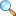# MTH103: Exploring Quantitative Skills

This course aims to develop the basic mathematical skills which ultimately enhance problem-solving skills using inductive and deductive reasoning, Polya's strategy, and sets. The basic concepts will be develop with applications form the real world such as algebraic models with equations, rates, ratios, and percentages will be discussed. Students will also explore linear models, including rectangular coordinates, functions, empowering them to analyze real-world problems with logical precision. By the course's end, students will have honed problem-solving, logical reasoning, and mathematical modeling abilities to tackle diverse challenges confidently.

#### Numeracy and Arithmetic:

Introduction to number systems, square roots, cube roots, highest common factors, lowest common multiples, visualizing fractions, decimals, systems of measurements, an overview of contributions of mathematicians, unit analysis as a problem-solving tool.

#### Problem Solving using Sets

Inductive and deductive reasoning, Problem Solving strategies including Pólya’s Problem-Solving Strategy and Problem solving using sets.

#### Functions:

Introduction to functions, rates of change, composition of functions, transformation of functions, absolute value function, inverse function, linear functions, polynomial functions, rational functions and applications related to functions.

#### Algebraic Models:

Linear equations, quadratic equations and their applications in social and economic problems.

#### Simple and Compound Interest:

Percentage, profit, loss, discount, taxation, and other scenarios involving percentage, simple and compound interest with applications.

#### Geometry:

Fundamentals of Geometry, Applications of Pythagorean theorem, Introduction to unit circles, trigonometric functions and inverse trigonometric functions, problem solving with geometry,

Please download PDF files of the notes given below. To view PDF files, there must be PDF Reader (Viewer) installed on your PC or mobile or smartphone. It can be downloaded from Software section or a user viewing on Android Smartphone may consider EBookDroid to view PDF on their smartphone.

• Slides 26-09-2023 | Download PDF |View OnlinePlease click on View Online to see inside the PDF.

1. Prove that 5 is divisor of 25.
2. Prove that -1 is divisor of 10.
3. Prove that 3 is not even number.
4. Prove that 2.2 is not even number.
5. Prove that 1 is not prime number.
6. Prove that 11 is prime number.
7. Prove that 8 is not prime number.
8. Prove that 8 is composite number.
9. Write the prime factorization of 60.
10. Write the prime factorization of 284.
11. Find the lowest number which is exactly divisible by 18 and 24.
12. A florist wants to arrange 24 boquets of flowers in different rows. Find out in how many ways he can arrange the bouquets with same number in each row.
13. A shopkeeper sells candles in packets of 12 and candle stands in packet of 8. What is the least number of candles and candle stands Areesha should buy so that there will be one candle for each candle stand.
14. Find the least number, which, when divided by 35, 56 and 91, leaves the same remainder of 7, respectively.
15. Two alarm clocks ring their alarms at regular intervals of 72 seconds and 50 seconds respectively. If they beep together at noon, at what time will they beep again together?
1. R. N. Aufmann, J. S. Lockwood, R. D. Natio and D. K. Clegg , Mathematical Thinking and Quantitative Reasoning(2008), Houghton Mifflin Company(New York).
2. Bennett, J. & Briggs, W. (2015). Using and understanding mathematics (6th Edition). Pearson Education, Limited.
3. Blitzer, R. (2014). Precalculus. (5th Edition). Pearson Education, Limited.